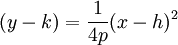SEARCH HOMEMath Central Quandaries & QueriesQuestion from San, a student: A movie theatre sells tickets for \$8.50 each. The manager is considering raising the prices but knows that for every 50 cents the price is raised, 20 fewer people go to the movies. The equation R= -40c^2+84c describes the relationship between the cost of the tickets, c dollars, and the amount of revenue, R dollars, that the theatre makes. What price should the theatre charge to maximize revenue? This question comes from my gr.11 corresponding study homework and I not yet solve it. Please help! Thank you, I will appreciate your help.Hi San.

The maximum revenue will occur at the top of the parabola that this quadratic equation describes.

Look at the quadratic equation you were given and change it into the form of a parabola:because that lets you just read off the position of the vertex (h, k).
The value of k is the maximum revenue.

Hope this helps,
Stephen La Rocque.Math Central is supported by the University of Regina and The Pacific Institute for the Mathematical Sciences.